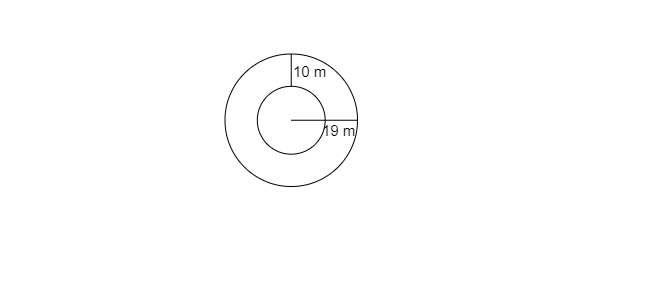# Find the circumference of the inner and the outer circles, shown in the adjoining figure? $(Take\ \pi=3.14)$"

The radius of the outer circle$= 19\ m$

Circumference of the outer circle$= 2\pi r$

$=2\times3.14\times19=3.14\times38\ m$

$=119.32\ m$

The radius of the inner circle

$=19\ m-10\ m=9\ m$

Circumference$=2\pi r=2\times3.14\times9$

$=56.52\ m$

Here the required circumferences are $56.52\ m$ and $119.32\ m$